[top.htm]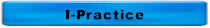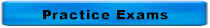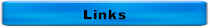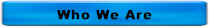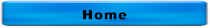Answers to More Water Treatment Questions

If a lime softening plant using a recarbonation process, where does this process occur?  Before or after lime softening?  Why is recarbonation used?  How is recarbonation accomplished?

• Recarbonation is used after the lime softening process

• Recarbonation is used to stabilize lime softened water and make it less depositing (scaling).

• CO2 dioxide is added to the lime softened water

Show the overall softening chemical equation for calcium bicarbonate removal using lime

Ca(HCO3)2 + Ca(OH)2  2CaCO3 + 2H2O

Show the chemical equation for carbon dioxide dissolving into water

CO2 + H2O H2CO3

In lime softening, which of the following is the most costly hardness to remove?  Why?

A.  Ca(HCO3)2

B.  MgCO3

C.  CaCl2

D.  MgSO4

D.  MgSO Because you have to add a source of carbonate alkalinity such as soda ash, then you have to remove magnesium carbonate hardness followed by having to remove the lime carbonate hardness.  This takes twice as much lime as removing calcium non-carbonate hardness.

The name and the chemical formula for the most common form of carbonate hardness is

Calcium bicarbonate, Ca(HCO3)2

What is the most common cation that forms hardness?  What is the second most common cation that forms hardness?

Calcium (Ca+2) is the most common cation that forms hardness

Magnesium (Mg+2) is the second most common cation that forms hardness

 Matching 1.  Chloride A.  NCl3 2.  Chlorine gas B.  HOCl 3.  Hydrochloric acid C.  OCl- 4.  Hypochlorite D.  Cl2 5.  Hypochlorus acid E.  NHCl2 6.  Monochloramine F.  HCl 7.  Dichloramine G.  NH2Cl 8.  Trichloramine H.  Cl-

1.  H

2.  D

3.  F

4.  C

5.  B

6.  G

7.  E

8.  A

What is the most common chloramine formed when chlorine reacts with ammonia in water?

A.  Monochloramines

B.  Dichloramines

CTrichloramines

DOrganic chloramines

E.  All of the above

Source:  USEPA 29 Questions on chloramines

The correct answer is A.  Monochloramines

The purpose of the USEPA Ground Water Rule is to reduce disease incidence associated with disease-causing microorganisms in

A.  Drinking water
B.  Non-point sources
C.  Storm water
D.  Wastewater

Source:  USEPA Ground Water Rule, http://www.epa.gov/safewater/disinfection/gwr/regulation.html

The correct answer is A.  Drinking Water

The USEPA Ground Water Rule becomes effective on

A. December 1, 2009

B. July 1, 2010

C. December 1, 2010

D. July 1, 2011

Source:  Iowa Department of Natural Resources' Water Supply Listserv - November 10, 2009

The correct answer is A.  December 1, 2009

Which of the following is another name for ion exchange softening?

A. Electrodialysis

B. Lime-soda softening

C. Nanofiltration

D. Zeolite softening

The correct answer is D.  Zeolite softening

Chlorine ton cylinders at room temperature will deliver gas at a maximum rate of

150 lb cylinders        Ton Containers

A.  10 pounds per day      100 pounds per day

B.  25 pounds per day      250 pounds per day

C.  40 pounds per day      450 pounds per day

D.  150 pounds per day    2000 pounds per day

The correct answer is C

Which of the following valves only permits flow to move in one direction?

A.  Butterfly

B.  Check

C.  Gate

D.  Plug

The correct answer is B

The specific gravity of a colloidal solid is very close to 1.  This means that the colloid

A.  Will float within an hour

B.  Will settle within an hour

C.  Won't settle or float

The correct answer is C

The specific gravity of settleable solid is

A.  1

B.  Greater than 1

C.  Less than 1

The correct answer is B

The specific gravity of a floatable solid is

A.  1

B.  Greater than 1

C.  Less than 1

The correct answer is C

The specific gravity of chlorine gas is

A.  1

B.  Greater than 1

C.  Less than 1

The correct answer is B

 Matching 1.    Calcium A.  C 2.    Carbon B.  S 3.    Chlorine C.  P 4.    Copper D.  K 5.    Fluorine E.  O 6.    Iron F.   Na 7.    Hydrogen G.  N 8.    Lead H.  Mg 9.    Magnesium I.    Ca 10.  Manganese J.   Cl 11.  Nitrogen K.  Cu 12.  Potassium L.   Mn 13.  Sodium M.   Fe 14.  Oxygen N.   H 15.  Phosphorus O.   Pb 16.  Sulfur P.   Fl

1.  I

2.  A

3.  J

4.  K

5.  P

6.  M

7.  N

8.  O

9.  H

10.  L

11.  G

12.  D

13.  F

14.  E

15.  C

16.  B

Which of the following is correct?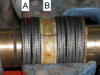A.  A is the follower and B is the lantern ring

B.  A is the lantern ring and B is a packing ring

C.  A is a packing ring and B is the lantern ring

D.  A is a packing ring and B is the follower

The correct answer is C.  A is a packing ring and B is the lantern ring

pH measures

A.  H+

B.  OH-

C.  O2

D.  Phosphorus

The correct answer is A.  H+  -- pH measures the hydrogen ion concentration

How do you know that your pump is cavitating?

A.  It doesn't sound right

B.  It runs hot

C.  It won't start

D.   All of the above

The correct answer is A.  It doesn't sound right.

A pump that's cavitating sounds like it's pumps rocks or gravel.

A chlorine atom is composed of

A.  Electrons

B.  Neutrons

C.  Protons

D.  All of the above

The correct answer is D.  All of the Above

Throttling the discharge valve on a centrifugal pump will the likely ______ temperature of the motor and ______ the temperature of the pump

A.  Increase, increase

B.  Increase, reduce

C.  Reduce, increase

D.  Reduce, reduce

The correct answer is C.  Reduce, increase

A, B, and D are incorrect because throttling the discharge valve on a centrifugal pump will the likely reduce temperature of the motor and increase the temperature of the pump

The chemical symbol for chlorine gas is

A.  Cl

B.  Cl-

C.  Cl2

D.  HOCl

The correct answer is C.  Cl2

A is incorrect because this is chemical symbol for chlorine not chlorine gas

B is incorrect because this is the chemical symbol for chloride

D is incorrect because this is the chemical formula for hypochlorite

Which of the following is the indicator used for pathogens?

A.  Amoebic dysentery

B.  E. coli

C.  Salmonella typhosa

D.  V. comma

The correct answer is B.  E. coli

A is incorrect because this is a pathogen, not an indicator

C is incorrect because this is a pathogen (cholera), not an indicator

D is incorrect because this is a pathogen (typhoid), not an indicator

Pump suction and discharge pressures are usually measured in

A.  gpm

B.  kWh

C.  mg/L

D.  psi

The correct answer is D.  psi

A is incorrect because this is a measure of flow

B is incorrect because this is a measure of energy used

C is incorrect because this is a measure of concentration

Which of the following is the correct formula for chlorine in water?

A.  Cl2 + H2O → HOCl + HCl

B.  NaOCl + H2O → HOCl + NaOH

C.  Ca(OCl)2 + 2H2O → 2HOCl + Ca(OH)2

D.  HOCl → H+ + OCl-

E.  All of the above

The correct answer is E.  All of the Above

Which of the following describes flocculation?

A.  Suspended solids that are too small to settle

B.  Quiescent conditions that allow settleable solids to settle

C.  Neutralizing charges

D.  Growth of smaller solids into larger masses that will settle

The correct answer is D.  Growth of smaller solids into larger masses that will settle

A is incorrect because this describes colloids

B is incorrect because this describes sedimentation

C is incorrect because this describes coagulation

Hypoxic zones are caused by high levels of

A.  Colloidal suspended solids

B.  Dissolved O2

C.  Nitrogen & phosphorus

D.  Pathogens

The correct answer is C.  Nitrogen & phosphorus

A is incorrect because high levels of colloidal suspended solids cause turbidity problems

B is incorrect because hypoxic zones have low or no dissolved oxygen

D is incorrect because a breakdown in the disinfection process would lead to high levels of pathogens

The PE for flow is _____ gpcd.

A.  7.48

B.  8.34

C.  150

D.  250

The correct answer is C.  150 gpcd

A is incorrect because this is the conversion factor from cubic foot to gallon

B is incorrect because this is the conversion factor between gallon and pounds

D is incorrect because a breakdown in the disinfection process would lead to high levels of pathogens

For questions or comments, call 515-313-1159

Home